### Vol 17, No 10 (2017) / Wang

How to co-add images? I. A new iterative method for image reconstruction of dithered observations

# How to co-add images? I. A new iterative method for image reconstruction of dithered observations

Wang Lei , Li Guo-Liang

Purple Mountain Observatory, Chinese Academy of Sciences, Nanjing 210008, China

† Corresponding author. E-mail: leiwang@pmo.ac.cn guoliang@pmo.ac.cn

Abstract: Abstract

By employing the previous Voronoi approach and replacing its nearest neighbor approximation with Drizzle in iterative signal extraction, we develop a fast iterative Drizzle algorithm, named fiDrizzle, to reconstruct the underlying band-limited image from undersampled dithered frames. Compared with the existing iDrizzle, the new algorithm improves rate of convergence and accelerates the computational speed. Moreover, under the same conditions (e.g. the same number of dithers and iterations), fiDrizzle can make a better quality reconstruction than iDrizzle, due to the newly discovered High Sampling caused Decelerating Convergence (HSDC) effect in the iterative signal extraction process. fiDrizzle demonstrates its powerful ability to perform image deconvolution from undersampled dithers.

Keywords: techniques: image processing;methods: observational;stars: imaging;planets and satellites: detection;gravitational lensing

## 1 Introduction

All imaging processes involve a limitation related to resolution of the equipment. In practice, the number of detectors is limited, thus the sampling is limited. Since spatial frequencies in an astronomical image are strongly limited by the optics of the telescope, the band is limited. For economical or other considerations, e.g. to cover a wide field in each exposure, the detector sampling sometimes cannot reach the Nyquist (or critical) sampling of the optics in a telescope. Therefore the detector often collects a set of undersampled data. In fact, an undersampled detector inevitably blurs the details in its sampling interval. This blurring effect is so-called aliasing. When the sampling process is executed by a CCD (or CMOS ) pixel matrix (via a digitizer), the effect of aliasing is expressed as pixelation.

In order to restore details lost in pixelation or aliasing, researchers have proposed increasing the sampling rate by increasing the number of exposures of the same field (but with different shifts, i.e. dithered frames). Thus the remaining question is how to reconstruct the signal from dithered frames. Many methods have been developed such as interlace and shift-and-add, which can obtain a high resolution result and reduce the pixelation to some extent, but these results are still far from excellent anti-aliasing. By taking advantage of interlace and shift-and-add, Fruchter & Hook () improve on the previous shift-and-add, named Drizzle. However, like the method mentioned before, Drizzle does not enhance the anti-aliasing function though it has a better performance than previous works in reducing noise and increasing accuracy. In fact, Drizzle generates a flux averaged image on a high resolution grid, thus producing a blurred, contrast reduced appearance. Based on a non-parametric method called kernel regression, which takes both the relative spatial and radiometric distances of nearby pixels into account, Takeda et al. () developed an improved method named super-Drizzle, which can reconstruct a high quality image compared with Drizzle. super-Drizzle has good performance in de-convolving the pixelation to some extent. However, super-Drizzle is more likely to be applied for image denoising and interpolation. It sensitively depends on the number of dithered frames and parameter selection. Thus it is difficult for super-Drizzle to obtain a higher contrast image than Drizzle when the dithers are not enough. By replacing the value of nearest neighbor with that of Drizzle in an iterative Voronoi approximation [initially developed by Werther (), Gröchenig & Strohmer ()] and introducing the oversampling - low pass filtering - interpolating process to the image co-adding procedure, Fruchter () upgraded the previous Voronoi approximation to yield iDrizzle. iDrizzle was developed largely for creating accurate images of objects with unresolved or nearly-unresolved components. With the help of iterative signal extraction and low pass filtering in the frequency domain, iDrizzle deconvolves the pixelation of undersampled features (with high signal-to-noise ratio, SNR) much better than super-Drizzle on small scales. However, iDrizzle has an oversampling, filtering and interpolation process, which dramatically increases the amount of computation.

In this paper, compared with the previous iDrizzle method, we improve on the effectiveness and computational speed, by introducing a program called fast iDrizzle or fiDrizzle. fiDrizzle can accelerate the computation and improve image quality. After describing the fiDrizzle algorithm and analyzing the theoretical aspects of its mechanism in Section 2, in Section 3 we illustrate the results to make a visual (Sect. 3.1) and a quantitative comparison (Sect. 3.2). We present the computational complexity analysis in Section 4 to show how the new algorithm can accelerate the computation. The algorithm’s dependency on the number of dithered frames and iterations is shown in Section 5. Finally, the discussion and conclusion are provided in the last section (Sect. 6).

## 2 The fiDrizzle algorithm

Drizzle is superior in computational speed. However, its intrinsic filter (pixelation) removes high frequency information on small scales. iDrizzle can reconstruct details on small scales to some extent at the cost of a very large amount of computation and a huge volume for the output file. In order to maintain the advantages of both Drizzle and iDrizzle and reduce their weaknesses, we develop fiDrizzle to improve image co-adding technology. The algorithm is described in the following steps:

After the iterations are complete, one can regard the final approximation as the best fitting image to the true one. Comparing with the previous oversampling - low pass filtering - interpolating process in the steps of iDrizzle, we basically (i) remove the low pass filtering process; (ii) directly co-add the observation frames to an (at most) critically sampled grid instead of an oversampled one; (iii) therefore, the final sin c interpolation is not necessary. The improvement accelerates the computation significantly. Compared with the Voronoi approximation, we just replace the nearest neighbor approximation with the Drizzle result at each iteration.

In order to figure out the difference between iDrizzle and fiDrizzle in theory, we will compare their result under the same resolution grid, e.g. critical sampling. It means that fiDrizzle directly samples the original image to critical output, while iDrizzle undergoes an oversampling - low pass filtering - interpolating process to obtain the same resolution. Let T be a signal in two dimensional space. After being undersampled by equipment, e.g. a telescope, one gets an image I with a dither shift ds (relative to the output target grid, including position and angle shift),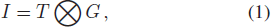where G represents all the combined effects from the true signal T to the equipment, such as seeing, PSF, pixelation, CCD distortion, etc. Symbol ⊗ represents the convolution operator. Now we resample I to an oversampling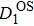and a critical sampling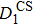, and in the meantime take the dither shift ds into account.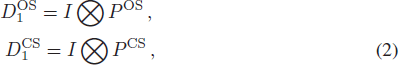where P OS or P CS is the resampling matrix which includes a pixelation effect. Also, P CS can be obtained by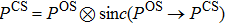, where sin c(P OSP CS) is lossless interpolation of the signal from oversampling to critical. Thereforeis equal to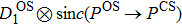. Hereoris the very result of Drizzle. Following the iterative reconstruction steps, we mimic the real observation by down sampling the target gridandto the I grid, thus getting the first approximations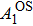and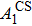to the original image I respectively. Then the difference (or residual image) from the original observation I can be expressed as: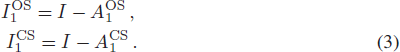Sincehas higher sampling than, correspondingly the residual image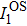possesses less power than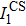, especially at the low frequency end. In an extreme case, if one oversamples the original image I to an infinitely high resolution grid,will keep an intact I, leading to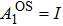, and thus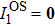. After the first iteration, we have the second approximations to the true image,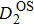and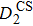: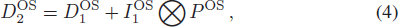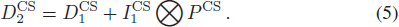Following the last step of iDrizzle, one may (sin c) interpolate the oversampled approximation to the critical sample grid with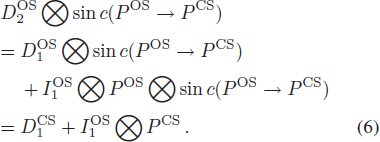Comparing Equation (6) to Equation (5), we find that for the same coarser (here critical) sampling case, iterative reconstruction on critical sampling gains more power (and hence more details) than that on oversampling, which means for the same number of iterations the rate of convergence for iterations is decelerated by the high sampling (here oversampling) case. We call this effect the High Sampling caused Decelerating Convergence (HSDC) 3 in the iterative signal extraction process. A simple simulation that serves as proof is provided in Appendix A of this paper. In fact, oversampling leaves more (original) pixelation effect in the final result than critical sampling. Therefore, fiDrizzle works more effectively in pixelation deconvolution than iDrizzle, even if we ignore the inserted filtering in the frequency domain in iDrizzle. In the next section, we provide more examples to test the HSDC effect and check the validity of the fiDrizzle algorithm.

## 3 The Results

We show visual and quantitative comparisons of all the three above mentioned image co-adding methods, Drizzle, iDrizzle and fiDrizzle, in the following subsections. According to sampling theory, if one wants to double the spatial resolution of the digital signal which is originally extracted from an analog signal, he (or she) should double the sampling frequency directly or double the number of observations (in different positions) but keep the sampling frequency unchanged, i.e. double the number of dithers (which is also the sense of dither). Therefore, in order to totally restore the signal from a set of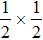undersampled observations, one should have at least four dithered frames to construct a critically-determined (or over-determined, which needs more dithers) system. Since this work mainly compares the co-adding methods on the critical sampling, in the following analysis, the systems are all over-determined for the critical sampling, but under-determined for the over-sampling, e.g. iDrizzle. We have checked a similar result when the system is over-determined for both critical and over sampling. Theoretically, an over-determined system can significantly reduce degeneracy from the effect of the random dithers (not well-placed dithered frames). In order to mimic a series of dithered frames, the true image is dithered to several undersampled frames by introducing random shifts, rotations and CCD geometric distortions of 0.1%.

3.1 A Visual Comparison

We use the well-known picture of Lena (with size 512 × 512 pixel, assuming this resolution meets the Nyquist (critical) sampling, i.e. the sampling frequency is at least twice the highest spatial frequency, which can be resolved by a telescope) to check the performance of the three co-adding methods. In Figure 1, the true image (left panel) is binned to five dithered frames with alower resolution (right panel, undersampled) than critical. Considering rotation and position shift, the dithered frames have a pixel size of at most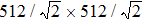(the critical sampling pixel size, i.e.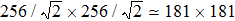original pixels), in which every pixel in the frame will not go beyond the region of the true image. In the dithered frame one can identify the effect of aliasing in regions with rich details, pixelation at the edge of the hat, blurred stripes in the body of the hat, and eyes and lips dimming. Such a mosaic-like image also exhibits loss of contrast and gray levels. In short, the effect of aliasing makes the image blurred and pixelized.Fig. 1 An image of Lena at different resolutions. One of the dithered images (right panel, which is usually regarded as the original input in image co-adding) haslower resolution than the true image (left panel). The effect of aliasing significantly smooths details on small scales. Details in 2 × 2 true image pixels (corresponding to one original pixel) are averaged out to a single value.

Figure 2 shows three reconstructions from the identical set of the (five) dithered frames: the upper left image is the true one, the upper right one is reconstructed by Drizzle, the lower left one is from the sin c interpolation of the oversampled 4 iDrizzle result and the lower right one is produced by this work, i.e. fiDrizzle. Following the strategy described in Appendix B, we choose a proper mask function with r f = 230 for the filtering steps in iDrizzle 5 . In the lower panels, both iDrizzle and fiDrizzle are applied in five iterations. Obviously, the Drizzle result (the upper right) is better than the dithered image as shown in the right panel of Figure 1. However, compared with the result from iDrizzle, Drizzle is not able to restore details at the level of a few pixels. There is an inherent filter (an averaging effect introduced by pixelation) convolved by the Drizzle mechanism, which results in high frequency information missing. That is the reason why the upper right image looks smoothed and blurred. Due to repeated signal extraction from the residual image, both iDrizzle and fiDrizzle co-add images much better than Drizzle does. Although the two lower images have a similar appearance, after a careful eyeball check, the right one looks sharper with higher contrast than the left. In addition, fiDrizzle generates more stripes 6 in the body of the hat, as well as sharper contrast in the hair and eyelashes, and thus yields better image quality than iDrizzle.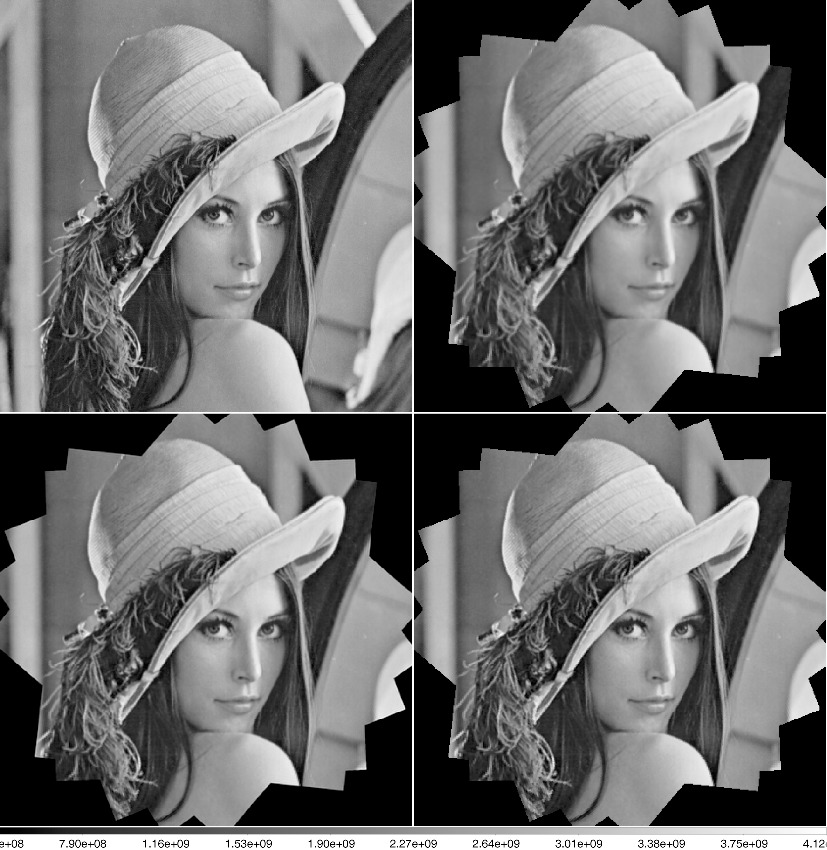Fig. 2 Reconstructions from three different methods. The upper left image is the true one. The upper right panel is from Drizzle, the lower left from iDrizzle and the lower right from fiDrizzle. Both iDrizzle and fiDrizzle are applied in five iterations. The three reconstructions have a 2 × 2 higher resolution than the original and thus have the same resolution as the true one.

Obvious differences are shown in the residual images (Fig. 3). In Figure 3, the upper left one is the same as in Figure 2, but the rest are differences between the reconstructions (generated by the three algorithms) and the true one. Three residual images are scaled to the same scope, then have the same color bar scale. For the portrait of Lena in the shadowed area, the more recognizable the figure is, the more signal it loses in image reconstruction. Evidently, the Drizzle algorithm loses lots of information, then shows a significant portrait in the residual image. iDrizzle misses a few details in high frequency. As a result, it leaves some features in regions with rich detail, e.g. hair, eyelashes and stripes in the hat. However, the residual from fiDrizzle is almost unrecognizable, which turns out to be the best fitting to the true case among the three results. If zooming in the lower panels and focusing on the sharp transition edges, one can find iDrizzle introduces a ringing artifact, which appears as ghosts near transients.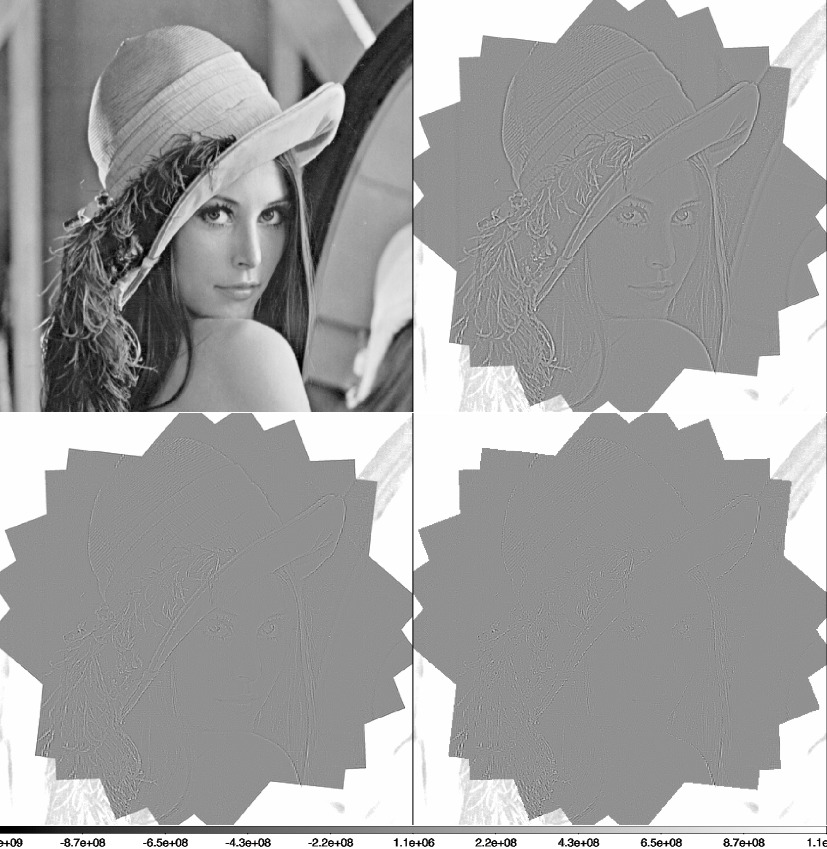Fig. 3 Residuals between the reconstructions and the true case (upper left panel). Upper right is for Drizzle, lower left for iDrizzle and lower right for fiDrizzle.

Furthermore, we try to figure out how the visual difference affects their power in the frequency domain. Here we introduce a reduced power spectrum (RPS, reduced to one dimension) to analyze the power left in the above three residual images. In order to avoid the regions in which pixels are not totally covered by all dithered frames, we select an all-covered area which is generally 1/4 times the true case, i.e. a pixel size of 256 × 256 (critical sampling pixel) and has the same center as the critical sample. We define the RPS as the radial power distribution in the fast Fourier transform (FFT) image of the all-covered area. Therefore, in the frequency direction the RPS has 128 pixels. In Figure 4, the black line stands for RPS of the true case. Other colors are the RPS (lower is better) of the three residuals between the true case and three reconstructions: green for Drizzle, blue for iDrizzle and red for fiDrizzle. Here one can find that most high frequency power is left in the three residuals. As expected, Drizzle is the worst one. Due to the oversampling mechanism and low-pass filtering, iDrizzle performs a little better than fiDrizzle at the high frequency end. However, iDrizzle loses much more power in low and medium frequencies than fiDrizzle because of the HSDC. This is the reason why Lena reconstructed by fiDrizzle has a high contrast level in Figure 2 and why the residual from iDrizzle in Figure 3 is still recognizable.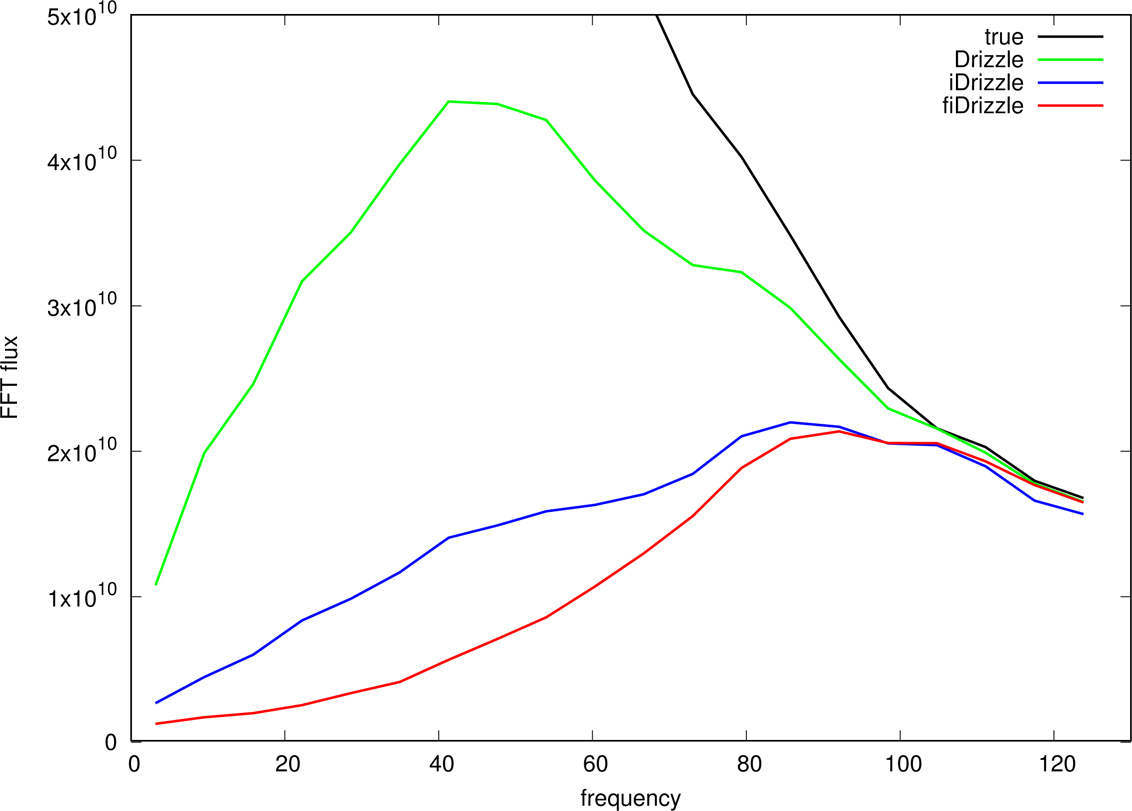Fig. 4 The RPS for residuals between reconstructions and the true image. The RPS for Drizzle is in green, iDrizzle in blue and fiDrizzle in red, while the black line is the RPS of the true image (NOT the residual).
3.2 The Quantitative Comparison

In weak gravitational lensing astronomy, people usually calculate the lensing effect by measuring the shapes of background galaxies and comparing them with a randomly oriented sample of galaxies. Its final result sensitively depends on the accuracy of shape measurement, which means a high fidelity image co-adding method can significantly improve the SNR of a weak lensing signal, thus enhancing the accuracy and reliability of the result. We extract a spiral galaxy image with a resolution of 512 × 512 from the Hubble Space Telescope (HST) observation HST_jclg03010_drc.fits at R.A. = 195.01238 deg and Dec = 28.023106 deg as the true picture to test. Then the true image is dithered to 10 frames (down sampled to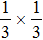lower resolution) by applying random shifts, rotations and CCD geometric distortions to mimic the undersampled dithered observations. Note that here iDrizzle has an oversampled grid with resolution 4 × 4 times the critical case. During the reconstruction, six iterations are executed in both iDrizzle and fiDrizzle. We plot the three kinds of reconstructions in Figure 5 with the same layout as in Figure 2. The color stands for the flux received by the pixels that were part of the observing equipment and is already scaled to the same scope. Also we show the residual plot in Figure 6 with the same representation and layout as in Figure 3. Similar to the result in Section 3.1, both iDrizzle and fiDrizzle recover better image quality than Drizzle. However, the visual difference between iDrizzle and fiDrizzle is not significant. So, we investigate the flux at the pixels that satisfy X = Y in the four panels of Figure 5.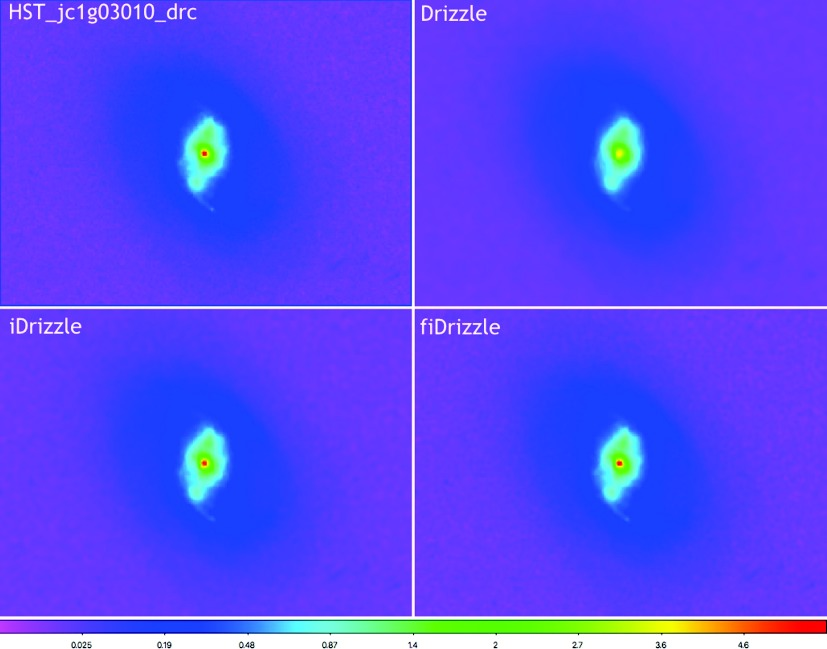Fig. 5 Another image reconstruction test, using HST data (with image center at R.A. = 195.01238 deg and Dec = 28.023106 deg). The layout of panels is similar to Fig. 2.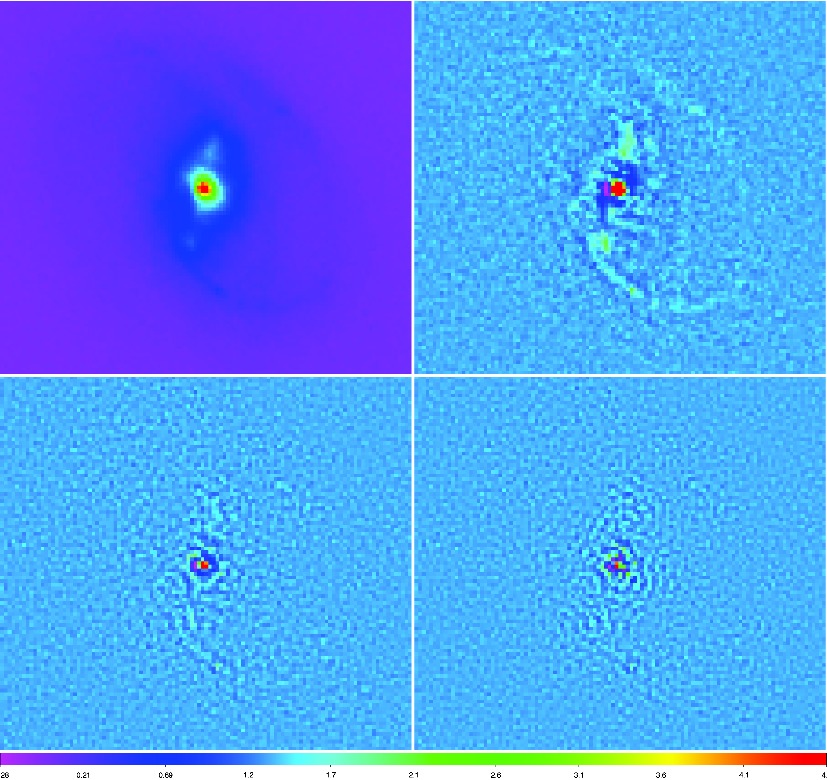Fig. 6 Residual maps for the HST image reconstructions. The layout of panels is the same as in Fig. 3.

In Figure 8, the flux profile, which is normalized to the flux of the central pixel of the true image, is plotted in the upper panel: the true one is in black, Drizzle in green, iDrizzle in blue and fiDrizzle in red. The lower panel shows the flux profile of the X = Y pixels in the residuals displayed in Figure 6. Here the size of 3 × 3 critically sampled pixels equals that of one original pixel. Evidently, fiDrizzle provides the best fitting result to the true case, especially at the center. Figure 7 shows a similar result as that in Figure 4: fiDrizzle is the best one in low and medium frequencies, but also leaves a few high frequency noises in the reconstruction. However, for shear measurement of weak gravitational lensing, low frequency information plays an important role. Following Hirata & Seljak (), the ellipticity of an object is defined as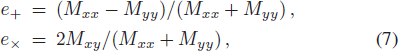where Mij represents the moments (see Hirata & Seljak () for details). The spin-2 tensor e = (e+, e×) is the so-called ellipticity tensor. In order to avoid the problem of divergence, a circular Gaussian weighting function with a weight radius of r w is convolved into the four images in Figure 5 before the measurement. We then plot the ellipticity tensor e of the source as a function of weight radius r w and show them in Figures 9 and 10. The color definition of line types is the same as that in Figures 8 and 4. Since only 39.3% of the weight is located in an area with radius r w, but 86.5% is in 2 × r w, here we use 2 × r w as the variable, which means if one faces a uniformly illuminated source, the flux from pixels in ≤ 2r w contributes 86.5% of the total to the measurement.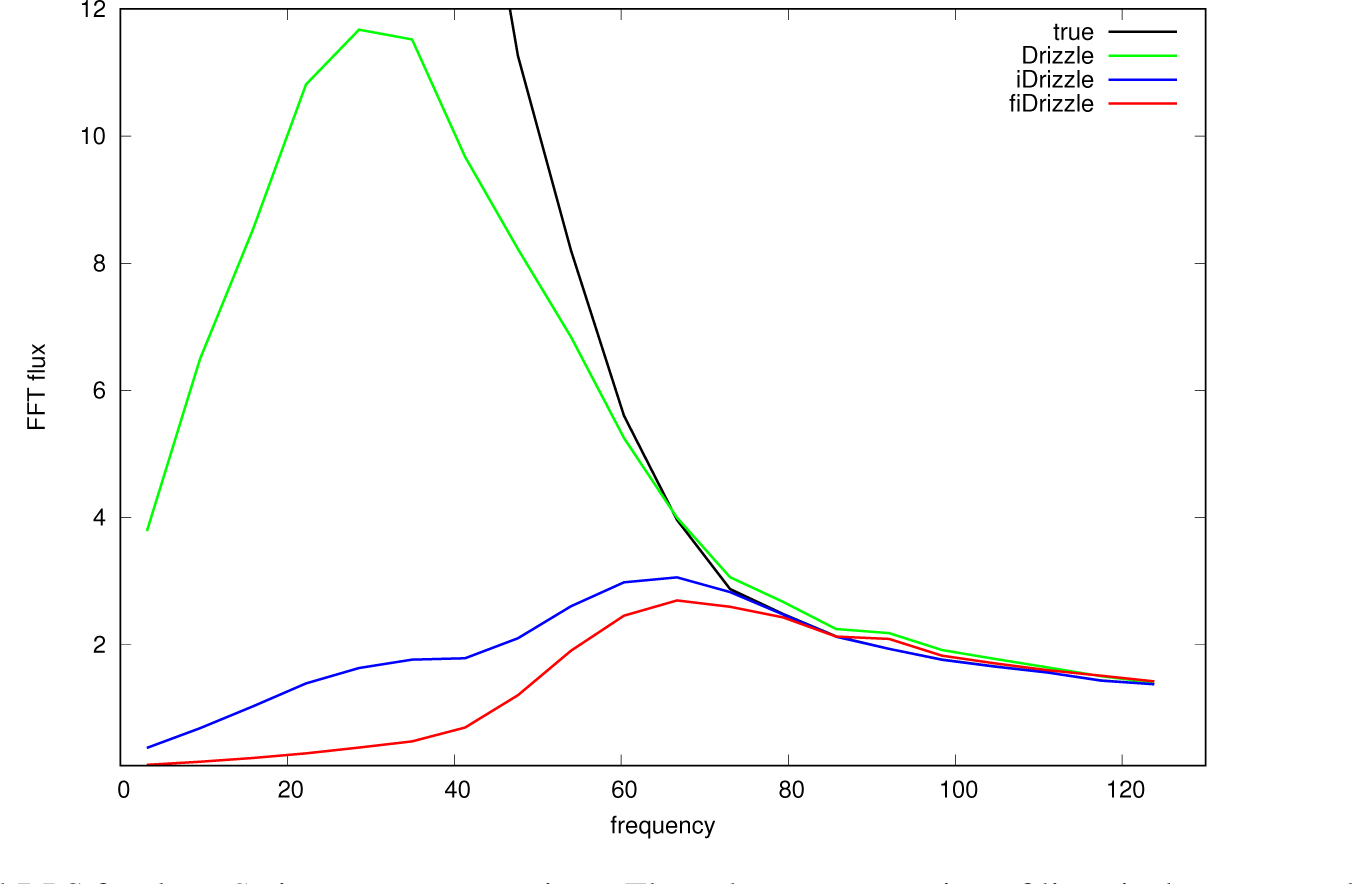Fig. 7 The residual RPS for the HST image reconstructions. The color representation of lines is the same as that in Fig. 4.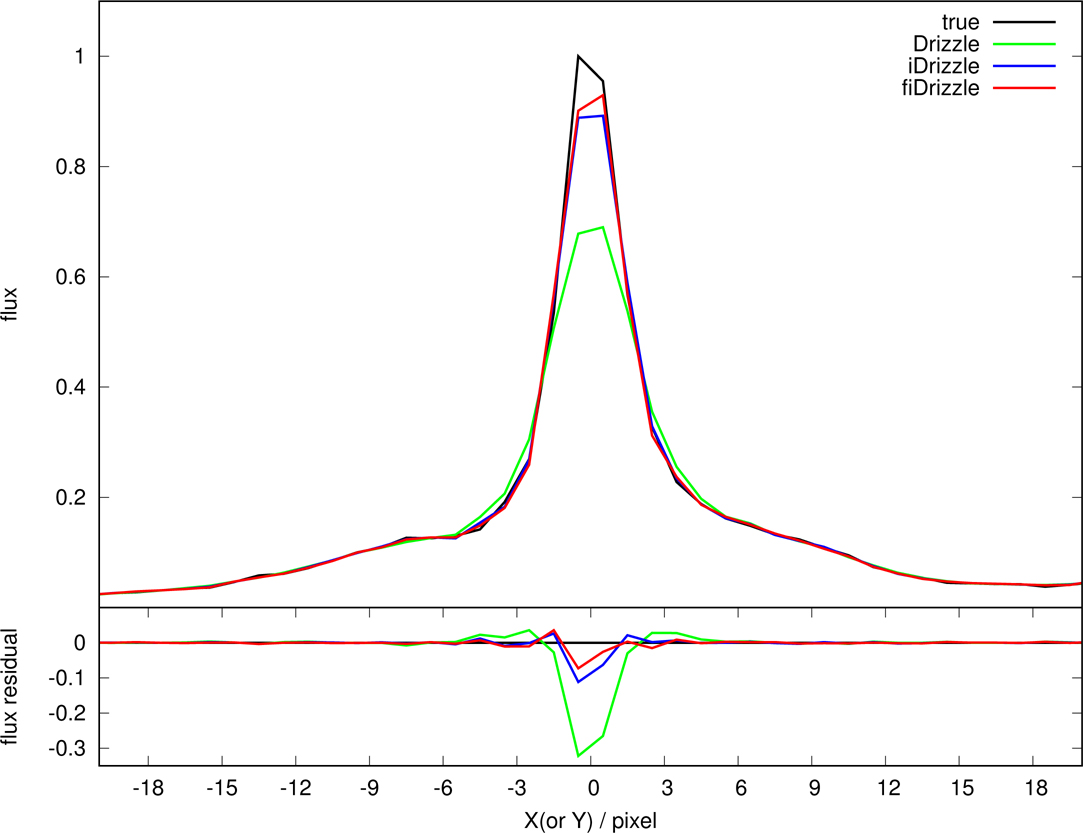Fig. 8 The flux and residual profile of the three reconstructions at the X = Y pixels. Lines have the same color definition as in Fig. 4. Note that the length of three pixels here corresponds to one original pixel.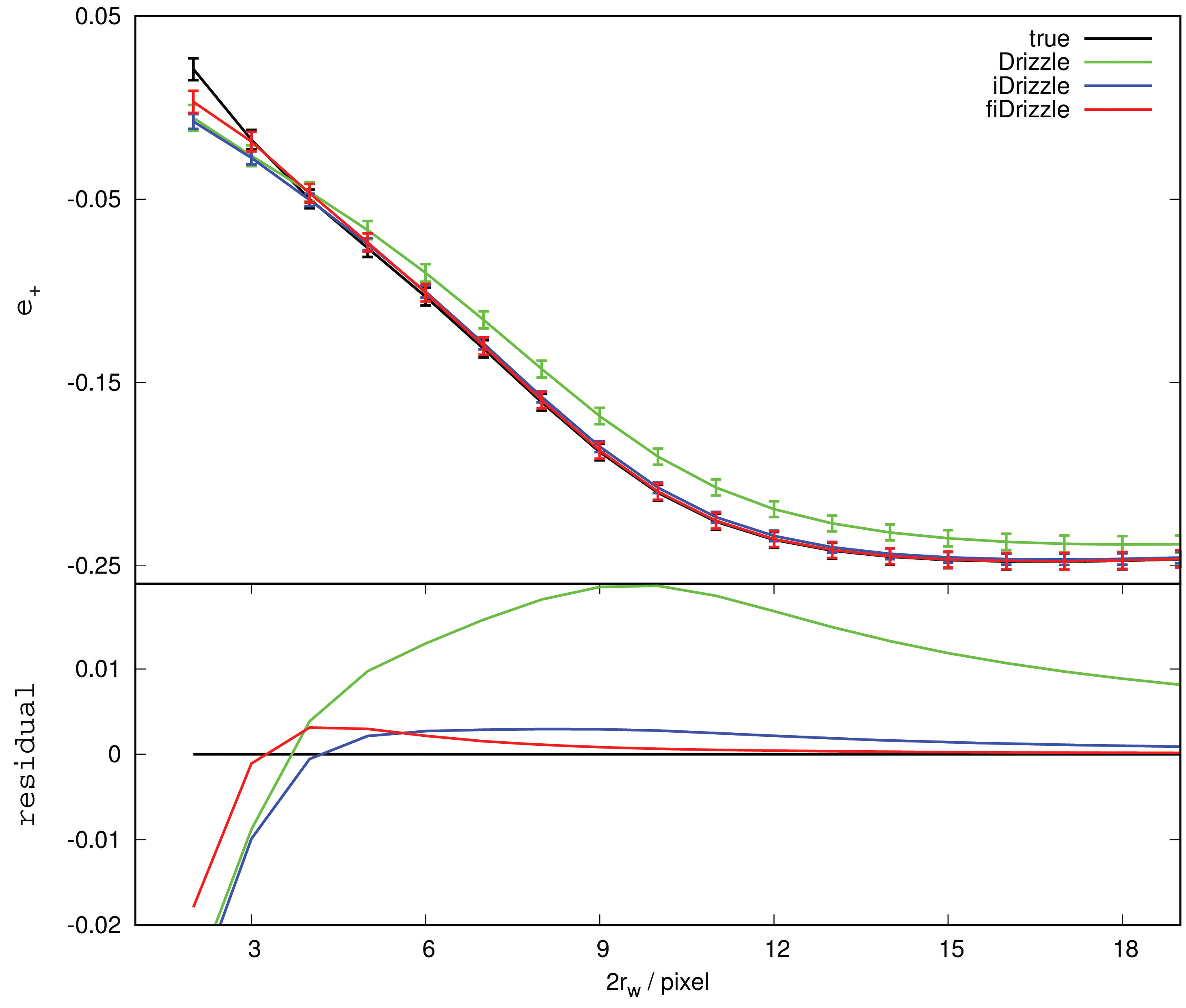Fig. 9 Measuring the ellipticity component e + as a function of weight radius r w. The error bars are estimated from the noise in the image. Line types are the same as those in Fig. 8.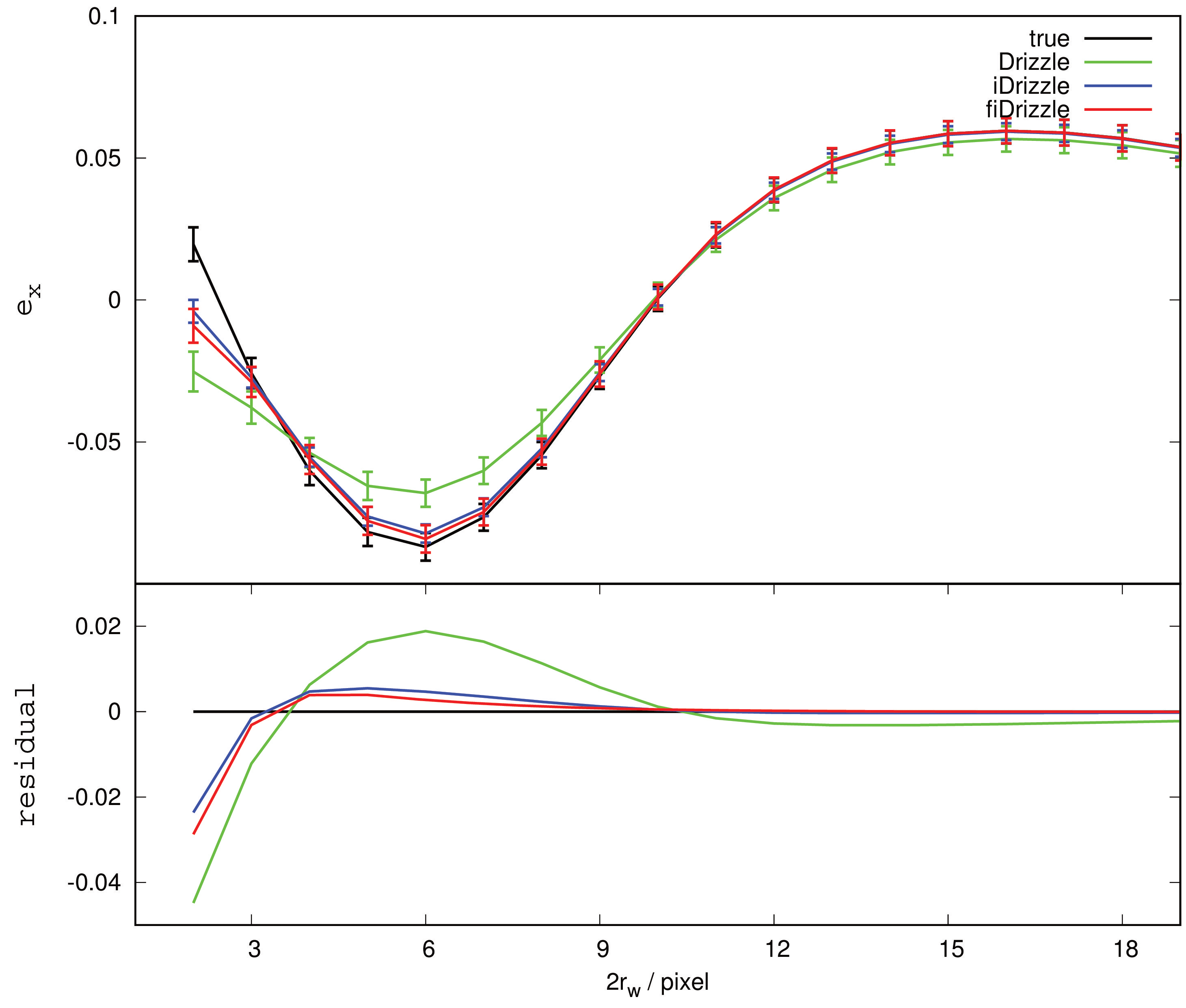Fig. 10 Similar to the profile in Fig. 9 but for another ellipticity component e ×.

From low to high frequency, Drizzle has no advantage compared with iDrizzle or fiDrizzle. This reflects how important pixelation deconvolution is in the image co-adding process. In the ellipticity measurement, power at low frequency determines the signal, while noise at high frequency mainly affects the scatter of the final result. In Figure 9, fiDrizzle behaves better than iDrizzle from small scales to large, and has the lowest systematic error among the three reconstructions at large scales. Note that gravitational lensing is very sensitive to systematic error. It turns out that the HSDC effect is the main reason that prevents iDrizzle from obtaining enough low frequency power and reducing the systematic bias in reconstruction. However, there is little difference between iDrizzle and fiDrizzle in the e × component of ellipticity in Figure 10. In this case, we find the disadvantage of fiDrizzle at high frequency does not badly affect the shear measurement because most of the high frequency noise is averaged out so as to be negligible at large scales.

## 4 The computational complexity

There is no doubt that Drizzle runs much faster than the other two algorithms because it does not need more iterations. The computation of each mimic observation [or blot program in Fruchter & Hook ()] is similar to that of Drizzle. Then fiDrizzle costs 2N times the computational complexity of Drizzle, which depends on the number of iterations N. In Figure 2, the true image has a size of 512 × 512 pixel, thus each original frame (observation) has a pixel size 7 of. Note that the workload of Drizzle depends not only on the number of total original pixels, 5 × 181 × 181, but also on the resolution of the output grid (for this example, the critical sampling is 2 × 2 times higher than the original grid). So, Drizzle costs at least T(n) = 5 × 181 × 181 × 2900 ≃ 470 000 000 computations 8 , and thus has a computational complexity O(2000n 2) (set n = 512). After five iterations, fiDrizzle has a total computational complexity O(20 000n 2), due to both Drizzle and the mimic observation process in each iteration. As for iDrizzle, there are three expenditures in amount of calculation:

First, iDrizzle requires an oversampled output grid which has a 2 × 2 (in this example) times higher resolution than the critical sampling. Then it results in a 4 times higher number of computations than fiDrizzle, i.e. a complexity O(80 000n 2).

Second, the workload of oversampled image FFT, smoothing and inverse FFT are, at least, T(n) = 5 × 2 × 10242 × 2 × log2(1024) ≃ 210 000 000, i.e. the total computational complexity is O(80n 2log2n ).

Third, the final sin c interpolation contributes an O(64n 2) computation complexity 9 .

Compared with fiDrizzle, iDrizzle is mainly delayed by the oversampling strategy. The CPU time consumed by the filtering process is about a few percent of the total when the size of an oversampled image is about 1024 × 1024. Combining the results above, we find that iDrizzle is not only decelerated in the rate of convergence (the HSDC effect) but also delayed in computational speed for the same reason — the oversampling strategy.

## 5 Dependency on the number of dithered frames and iterations

In this section, we discuss how fiDrizzle or iDrizzle depends on the number of dithered frames K and iterations N. We run fiDrizzle to reconstruct the picture of Lena for one, two, four and five frames. Each reconstruction is performed in five iterations. N = 5 is a tradeoff between signal extraction and artifact reduction, such as the ringing artifact near sharp transitions, which is introduced by low pass filtering but is enhanced by successive iterations. The residual (the difference between the fiDrizzle reconstructions and the true image) plot is shown in Figure 11; the upper left panel is for one dither, the upper right panel is for two dithers, four frames are in the lower left panel and five frames are in the lower right. Obviously, the residuals are significantly reduced when we increase the number of co-added frames. Moreover, a strong argument can also be found in the RPS plot of the residuals in Figure 12. In order to compare fiDrizzle (in solid lines) with previous work, we also plot the results of iDrizzle (in dotted lines, with the same filter as Figure 2) in the RPS figure. Note that the color representation is totally different from the above figures: reconstructions for one, two, four and five frames are in purple, red, blue and black respectively. Figure 12 shows that the quality of reconstruction strongly depends on the number of dithers when K is small. While the degree of this dependence decreases as K increases, one can also find that compared with fiDrizzle the advantage of iDrizzle on the high frequency end is diminished as the number of dithers K increases.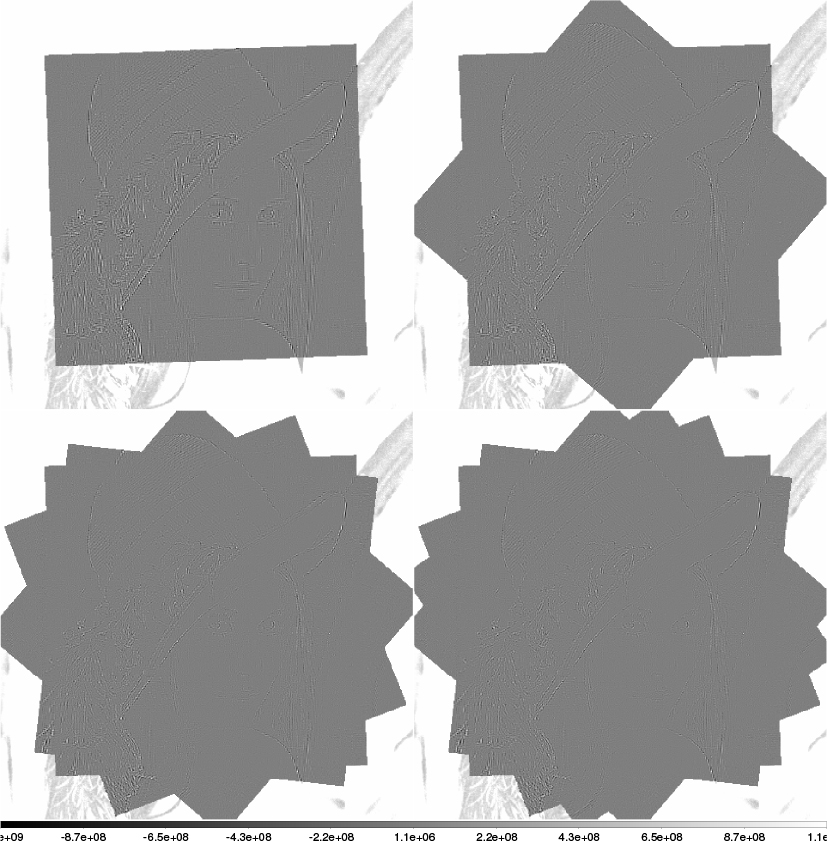Fig. 11 Residuals from fiDrizzle for different numbers of dithers. The upper left panel is for one dither, the upper right panel for two dithers, four frames are in lower left panel and five frames are in the lower right.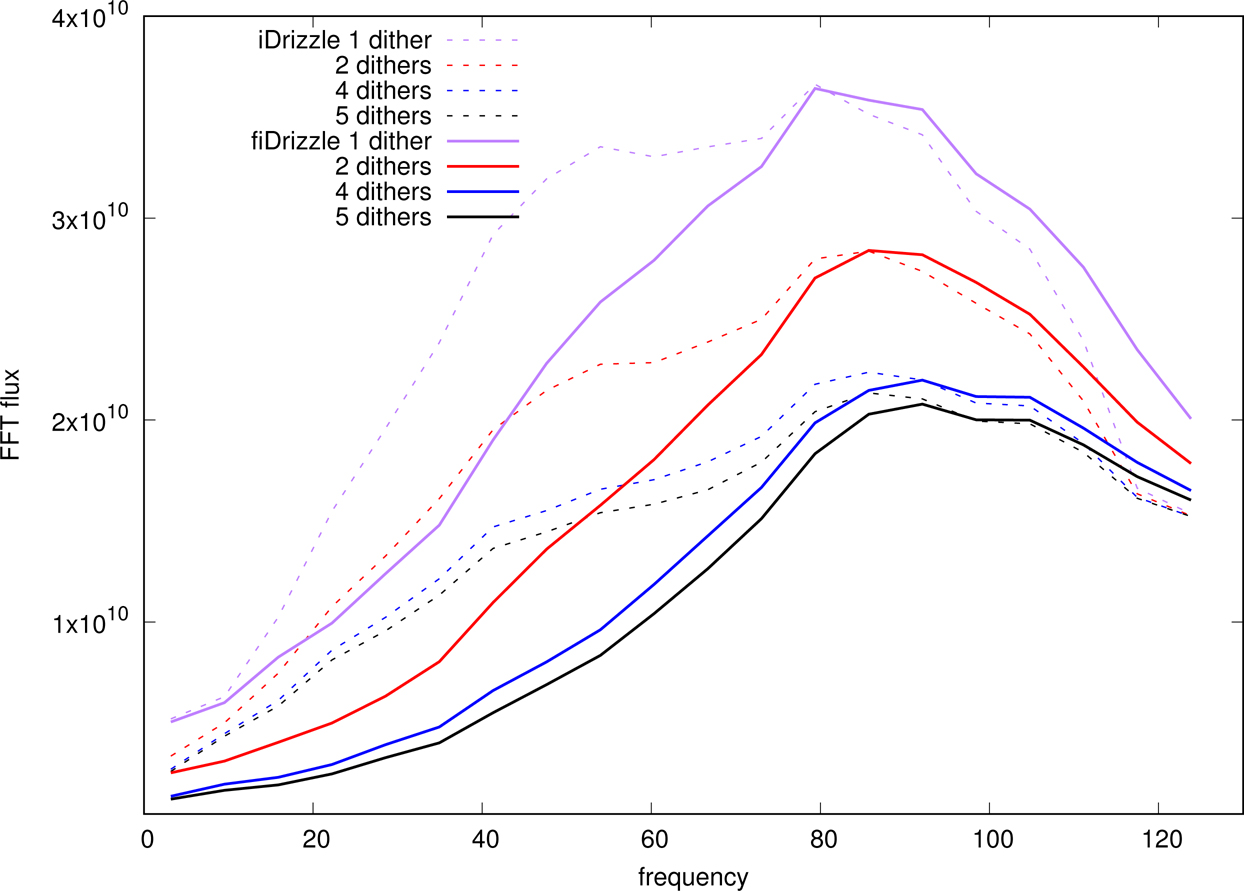Fig. 12 The residual RPS of iDrizzle and fiDrizzle for different numbers of dithers. The reconstructions for one, two, four and five frames are in purple, red, blue and black respectively. fiDrizzle is shown in solid lines, while iDrizzle is in dotted lines.

Now we fix the number of dithers K to be 5 and change the number of iterations N = 0, 1, 3, 5 for both fiDrizzle and iDrizzle. Here we only show the fiDrizzle reconstructed picture of Lena in Figure 13; the upper left panel is for zero iterations, namely the Drizzle result, the upper right panel is for one iteration, three iterations are executed in the lower left panel and five iterations are in the lower right. The efficiency of signal extraction is very high at the beginning several iterations, which results in the portrait of Lena vanishing quickly. Sufficient evidence is shown in Figure 14. Reconstructions for zero, one, three and five iterations are in purple, red, blue and black respectively (solid lines). As before, we also illustrate the results from iDrizzle with corresponding iterations and colors, but in dotted lines. Note that for the case of zero iterations with iDrizzle, we perform the filtering process after the first Drizzle step is complete, with no more signal extraction steps. From Figure 14, due to the HSDC effect we find that fiDrizzle converges more effectively than iDrizzle in low and medium frequencies. The low frequency difference between solid and dotted (same color) lines becomes large as the number of iterations N increases.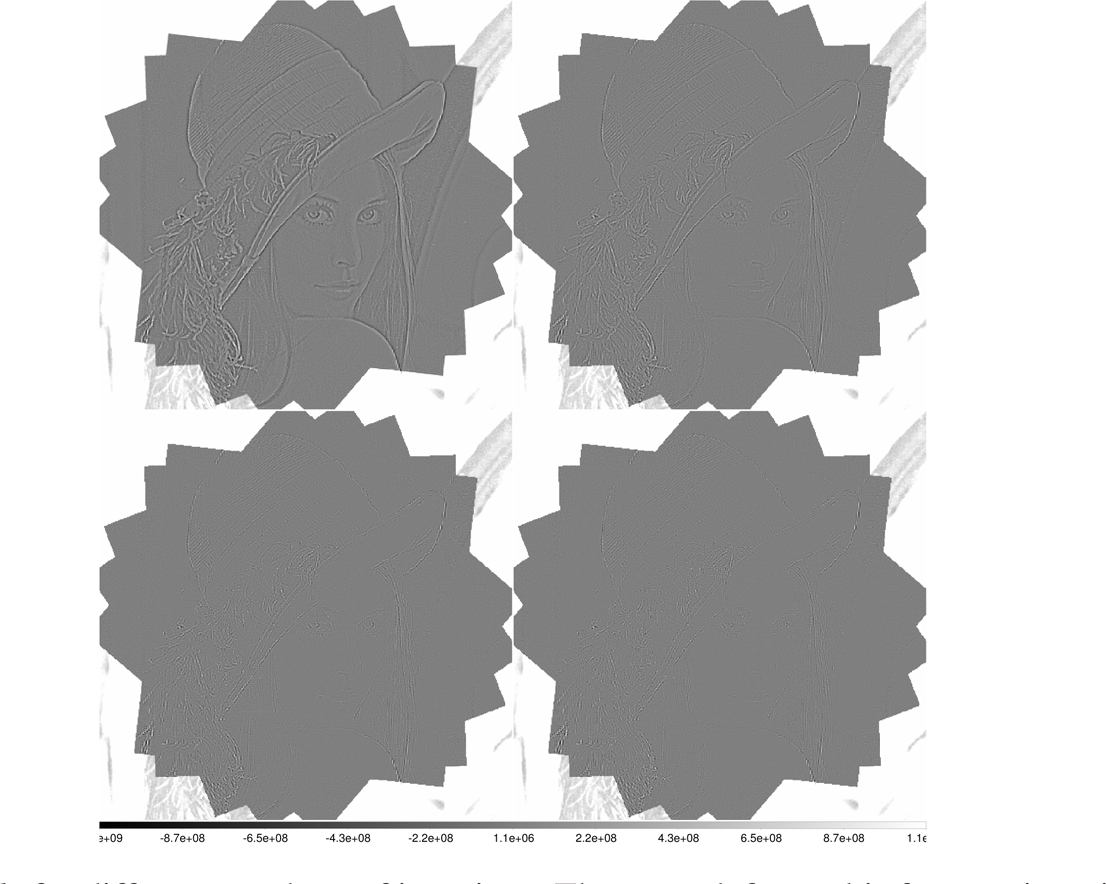Fig. 13 Residuals from fiDrizzle for different numbers of iterations. The upper left panel is for zero iterations, the upper right panelis for one iteration, three iterations are executed in the lower left panel and five iterations are in the lower right.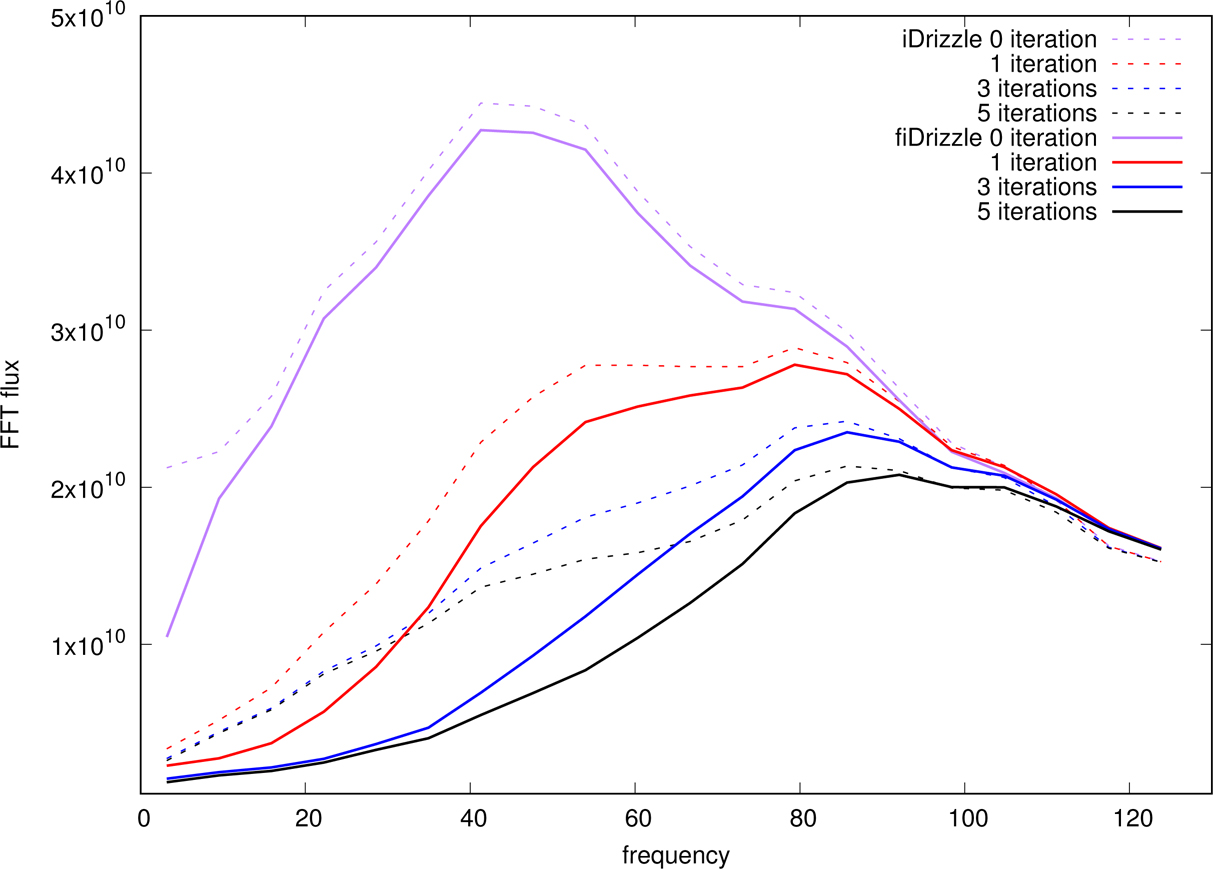Fig. 14 The residual RPS of iDrizzle (dotted lines) and fiDrizzle (solid lines) for different numbers of iterations. Reconstructions for zero, one, three and five iterations are in purple, red, blue and black respectively.

## 6 Discussion and conclusions

The oversampling - low pass filtering - interpolating process is a standard industry practice for improving the SNR in analog to digital (A/D) signal transition and extraction. Naturally, this process is adopted by the previous work related to iDrizzle. Of course, there is no problem if one initially wants an oversampled reconstruction or the process does not involve iterative signal extraction from the residuals. However, the oversampling - low pass filtering - interpolating process and iterative signal extraction coexist in iDrizzle, which inevitably encounters the HSDC effect. As a result, compared with fiDrizzle’s direct sampling to the critical case, iDrizzle not only costs more computational resources but also converges ineffectively which leads to an inadequate reconstruction for low frequency signals and, eventually, affects the systematic errors in weak lensing shear measurement as described in Section 3.2. Briefly, in this work we reach some goals:

We discover the HSDC effect in the iterative signal extraction process and mathematically prove its existence.

For the same number of iterations, fiDrizzle converges more effectively than iDrizzle, especially at low and medium frequencies, thus obtaining a better quality reconstruction.

Instead of oversampling the frames to a high resolution grid (iDrizzle), fiDrizzle directly samples the dithers to the critical resolution and omits the filtering and interpolation procedures, which finally saves more computational resources.

As mentioned before, iDrizzle can generate accurate images of objects with unresolved or nearly-unresolved components. fiDrizzle inherits this function as well if one co-adds the dithers in an oversampled grid at the very beginning. However, any features less than the scale of the maximum angular resolution of the optics are unbelievable, which are smoothed by the filter in iDrizzle, but retained in fiDrizzle. Nevertheless, it does not affect the photometry in both iDrizzle and fiDrizzle. In that sense, we do not include a comparison of unresolved features in the paper.

It is worth mentioning that compared with iDrizzle, fiDrizzle displays its lack of reconstruction accuracy at the high frequency end. In our upcoming work, on one hand we improve fiDrizzle to enable it to restore a part of details at the high frequency, and on the other hand, we develop a totally new image co-adding method called Tessellated Simple Surface Fitting (TSSF), which can effectively balance pixelation deconvolution and noise reduction.

In the future, many new telescopes will start astronomical observation, e.g. NASA’s Wide Field Infrared Survey Telescope (WFIRST), the European Space Agency (ESA)’s Euclid, the National Science Foundation (NSF) funded Large Synoptic Survey Telescope (LSST) and the Chinese Space Station optical Telescope (CSST). Huge amounts of imaging data will be generated by those telescopes. How to effectively and efficiently process these data will be an urgent requirement. We believe that the fiDrizzle algorithm has some advantages and can make some contributions in astronomical image processing as long as undersampled dithers exist.

 Fruchter A. S. Hook R. N. 2002 PASP 114 144 Fruchter A. S. 2011 PASP 123 497 Gröchenig K. Strohmer T. 2001 Nonuniform Sampling: Theory and Practice Springer Hirata C. Seljak U. 2003 MNRAS 343 459 Takeda H. Farsiu S. Christou J. Milanfar P. 2006 The Advanced Maui Optical and Space Surveillance Technologies Conference E27 Werther, T. 1999, Reconstruction from Irregular Samples with Improved Locality http://citeseerx.ist.psu.edu/viewdoc/summary?doi=10.1.1.28.402
Cite this article: Wang Lei, Li Guo-Liang. How to co-add images? I. A new iterative method for image reconstruction of dithered observations. Cancer Biol Med. 2017; 10:100.

### Refbacks

• There are currently no refbacks.